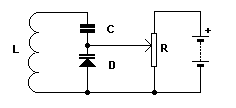Electronic Circuits and Tutorials
Tutorials
Dictionaries
Projects
Discover
Home > Electronics Tutorial > The Diode Tutorial > Varicap Diode Tutorial
The Diode - Electronic Tutorials

# Varicap Diode TutorialWhen the junction diode is reverse biased, the insulating barrier widens.

The higher the reverse voltage the wider the barrier becomes.

The barrier forms the dielectric, of variable width, of a capacitor.

The N and P type cathode and anode are the two plates of the capacitor.

In the diagram, the diode and coil form a resonant circuit. The capacitance of the diode, and thereby the resonant frequency, is varied by means of the potentiometer controlling the reverse voltage across the varicap.

The capacitor prevents the coil shorting out the voltage across the potentiometer